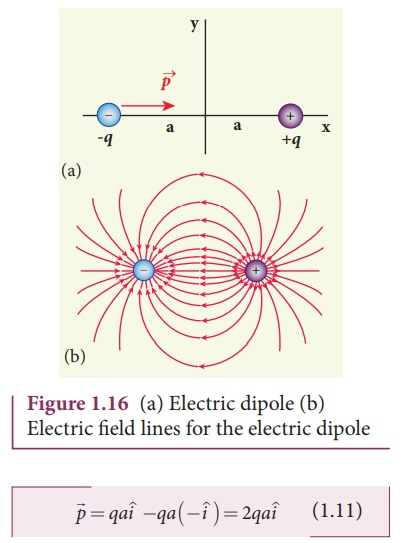Home | | Physics 12th Std | Electric dipole

# Electric dipole

Two equal and opposite charges separated by a small distance constitute an electric dipole.

Electric dipole

Two equal and opposite charges separated by a small distance constitute an electric dipole. In many molecules, the centers of positive and negative charge do not coincide. Such molecules behave as permanent dipoles. Examples: CO, water, ammonia, HCl etc.

Consider two equal and opposite point charges (+q, -q) that are separated by a distance 2a as shown in Figure 1.16(a). The electric dipole moment is defined asHere+ is the position vector of +q from the origin and- is the position vector of -q from the origin. Then, from Figure 1.16 (a),The electric dipole moment vector lies along the line joining two charges and is directed from –q to +q. The SI unit of dipole moment is coulomb meter (Cm). The electric field lines for an electric dipole are shown in Figure 1.16 (b).

·        For simplicity, the two charges are placed on the x-axis. Even if the two charges are placed on y or z-axies, dipole moment will point from –q to +q. The magnitude of the electric dipole moment is equal to the product of the magnitude of one of the charges and the distance between them, || = 2qa.

·        Though the electric dipole moment for two equal and opposite charges is defined, it is very general. It is possible to define and calculate the electric dipole moment for a single charge, two positive charges, two negative charges and also for more than two charges.

For a collection of n point charges, the electric dipole moment is defined as follows:wherei is the position vector of charge qi from the origin.

EXAMPLE 1.10

Calculate the electric dipole moment for the following charge configurations.Solution

Case (a) The position vector for the +q on the positive x-axis is ai and position vector for the +q charge the negative x axis is -a i ^ . So the dipole moment is,Case (b) In this case one charge is placed at the origin, so its position vector is zero. Hence only the second charge +q with position vector ai contributes to the dipole moment, which is= qa.

From both cases (a) and (b), we can infer that in general the electric dipole moment depends on the choice of the origin and charge configuration. But for one special case, the electric dipole moment is independent of the origin. If the total charge is zero, then the electric dipole moment will be the same irrespective of the choice of the origin. It is because of this reason that the electric dipole moment of an electric dipole (total charge is zero) is always directed from –q to +q, independent of the choice of the origin.

Case (c). Note that in this case p is directed from -2q to +q.

Case (d)The water molecule (H2O) has this charge configuration. The water molecule has three atoms (two H atom and one O atom). The centers of positive (H) and negative (O) charges of a water molecule lie at different points, hence it possess permanent dipole moment. The O-H bond length is 0.958 × 10-10 m due to which the electric dipole moment of water molecule has the magnitude p = 6.1 x 10-30 Cm. The electric dipole momentis directed from center of negative charge to the center of positive charge, as shown in the figure.Tags : Electrostatics , 12th Physics : Electrostatics
Study Material, Lecturing Notes, Assignment, Reference, Wiki description explanation, brief detail
12th Physics : Electrostatics : Electric dipole | Electrostatics